Fundamental Parameters Of Traffic Flow - 2

# Fundamental Parameters Of Traffic Flow - 2 - Notes | Study Transportation Engineering - Civil Engineering (CE)

 1 Crore+ students have signed up on EduRev. Have you?

Time mean speed (vt)

As noted earlier, time mean speed is the average of all vehicles passing a point over a duration of time. It is the simple average of spot speed. Time mean speed vt is given by,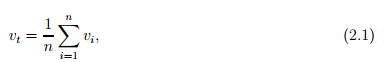where vi is the spot speed of ith vehicle, and n is the number of observations. In many speed studies, speeds are represented in the form of frequency table. Then the time mean speed is given by,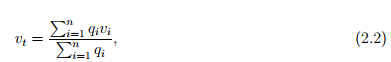where qi is the number of vehicles having speed vi , and n is the number of such speed categories.

Space mean speed (vs)

The space mean speed also averages the spot speed, but spatial weightage is given instead of temporal. This is derived as below. Consider unit length of a road, and let vi is the spot speed

of i th vehicle. Let tis the time the vehicle takes to complete unit distance and is given by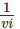. If there are n such vehicles, then the average travel time ts is given by,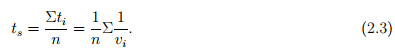If tav is the average travel time, then average speed vs =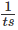. Therefore, from the above equation,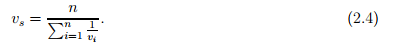This is simply the harmonic mean of the spot speed. If the spot speeds are expressed as a frequency table, then,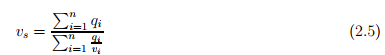where qi vehicle will have vi speed and ni is the number of such observations

Numerical Example

If the spot speeds are 50, 40, 60, 54 and 45, then find the time mean speed and space mean speed.

Solution Time mean speed vt is the average of spot speed. Therefore,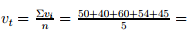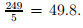Space mean speed is the harmonic mean of spot speed. Therefore,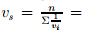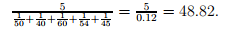Numerical Example

The results of a speed study is given in the form of a frequency distribution table. Find the time mean speed and space mean speed.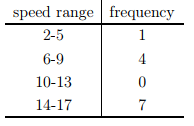Solution The time mean speed and space mean speed can be found out from the frequency table given below. First, the average speed is computed, which is the mean of the speed range. For example, for the first speed range, average speed, vi = 2+5 2 = 3.5 seconds. The volume of flow qi for that speed range is same as the frequency. The terms vi .qi and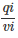are also tabulated,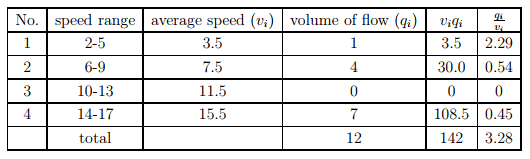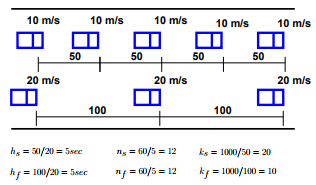Figure 2:1: Illustration of relation between time mean speed and space mean speed

and their summations given in the last row. Time mean speed can be computed as,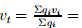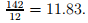Similarly, space mean speed can be computed as,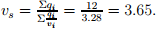Illustration of mean speeds

In order to understand the concept of time mean speed and space mean speed, following illustration will help. Let there be a road stretch having two sets of vehicle as in figure 2:1. The first vehicle is traveling at 10m/s with 50 m spacing, and the second set at 20m/s with 100 m spacing. Therefore, the headway of the slow vehicle hs will be 50 m divided by 10 m/s which is 5 sec. Therefore, the number of slow moving vehicles observed at A in one hour ns will be 60/5 = 12 vehicles. The density K is the number of vehicles in 1 km, and is the inverse of spacing. Therefore, Ks = 1000/50 = 20 vehicles/km. Therefore, by definition, time mean speed vt is given by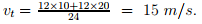Similarly, by definition, space mean speed is the mean of vehicle speeds over time. Therefore,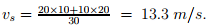This is same as the harmonic mean of spot speeds obtained at location A; ie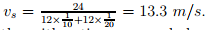It may be noted that since harmonic mean is always lower than the arithmetic mean, and also as observed, space mean speed is always lower than the time mean speed. In other words, space mean speed weights slower vehicles more heavily as they occupy the road stretch for longer duration of time. For this reason, in many fundamental traffic equations, space mean speed is preferred over time mean speed.

Relation between time mean speed and space mean speed

The relation between time mean speed(vt) and space mean speed(vs) is given by the following relation: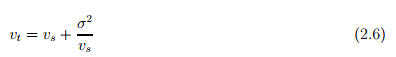where,σ 2 is the standard deviation of the spot speed. The derivation of the formula is given in the next subsection. The standard deviation(σ 2) can be computed in the following equation: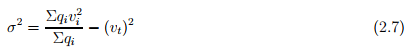where,qi is the frequency of the vehicle having vspeed.

Derivation of the relation The relation between time mean speed and space mean speed can be derived as below. Consider a stream of vehicles with a set of sub-stream flow q1, q2, . . . qi , . . . qn having speed v1,v2, . . . vi , . . . vn. The fundamental relation between flow(q), density(k) and mean speed vs is,

q = k × vs                                                                                                         (2.8)

Therefore for any sub-stream qi , the following relationship will be valid.

qi = ki × vi                                                                                                        (2.9)

The summation of all sub-stream flows will give the total flow q:

Σqi = q.                                                                                                            (2.10)

Similarly the summation of all sub-stream density will give the total density k.

Σki = k.                                                                                                             (2.11)

Let fdenote the proportion of sub-stream density ki to the total density k,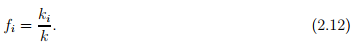Space mean speed averages the speed over space. Therefore, if ki vehicles has vi speed, then space mean speed is given by,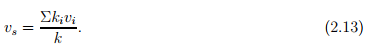Time mean speed averages the speed over time. Therefore,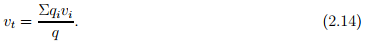Substituting 2.9, vt can be written as,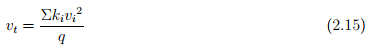Rewriting the above equation and substituting 2.12, and then substituting 2.8, we get,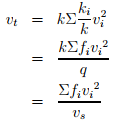By adding and subtracting vs and doing algebraic manipulations, vt can be written as,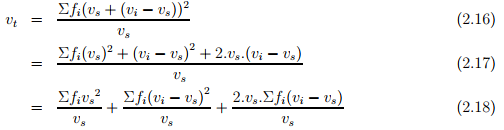The third term of the equation will be zero because Σfi(vi − vs) will be zero, since vs is the mean speed of vi . The numerator of the second term gives the standard deviation of vi . Σfi by definition is 1.Therefore,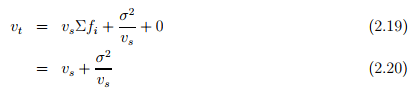Hence, time mean speed is space mean speed plus standard deviation of the spot speed divided by the space mean speed. Time mean speed will be always greater than space mean speed since standard deviation cannot be negative. If all the speed of the vehicles are the same, then spot speed, time mean speed and space mean speed will also be same.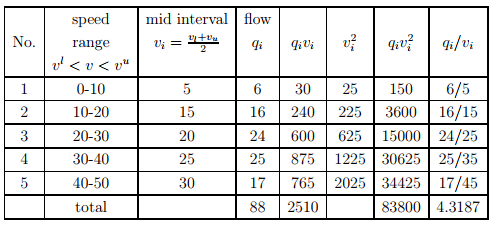Numerical Example

For the data given below,compute the time mean speed and space mean speed. Also verify the relationship between them. Finally compute the density of the stream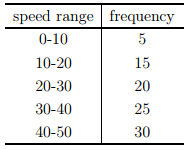Solution The solution of this problem consist of computing the time mean speed vt =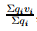, space mean speed vs =, verifying their relation by the equation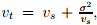and using this to compute the density. To verify their relation, the standard deviation also need to be computed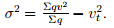For convenience,the calculation can be done in a tabular form as shown in table 2.5.1.

The time mean speed(vt) is computed as: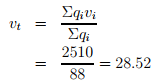The space mean speed can be computed as: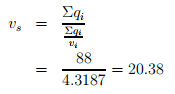The standard deviation can be computed as: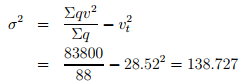The time mean speed can also vt can also be computed as: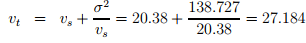The density can be found as: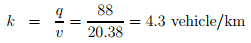Fundamental relations of traffic flow

The relationship between the fundamental variables of traffic flow, namely speed, volume, and density is called the fundamental relations of traffic flow. This can be derived by a simple concept. Let there be a road with length v km, and assume all the vehicles are moving with v km/hr.(Fig 2:2). Let the number of vehicles counted by an observer at A for one hour be n1. By definition, the number of vehicles counted in one hour is flow(q). Therefore,

n1 = q.                                                         (2.21)

Similarly, by definition, density is the number of vehicles in unit distance. Therefore number of vehicles n2 in a road stretch of distance v1 will be density × distance.Therefore,

n2 = k × v.                                                   (2.22)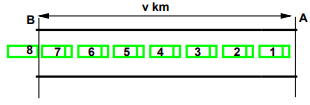Figure 2:2: Illustration of relation between fundamental parameters of traffic flow

Since all the vehicles have speed v, the number of vehicles counted in 1 hour and the number of vehicles in the stretch of distance v will also be same.(ie n1 = n2). Therefore,

q = k × v.                                                  (2.23)

This is the fundamental equation of traffic flow. Please note that, v in the above equation refers to the space mean speed will also be same

Fundamental diagrams of traffic flow

The relation between flow and density, density and speed, speed and flow, can be represented with the help of some curves. They are referred to as the fundamental diagrams of traffic flow. They will be explained in detail one by one below.

Flow-density curve

The flow and density varies with time and location. The relation between the density and the corresponding flow on a given stretch of road is referred to as one of the fundamental diagram of traffic flow. Some characteristics of an ideal flow-density relationship is listed below:

1. When the density is zero, flow will also be zero,since there is no vehicles on the road.

2. When the number of vehicles gradually increases the density as well as flow increases.

3. When more and more vehicles are added, it reaches a situation where vehicles can’t move. This is referred to as the jam density or the maximum density. At jam density, flow will be zero because the vehicles are not moving.

4. There will be some density between zero density and jam density, when the flow is maximum. The relationship is normally represented by a parabolic curve as shown in figure 2:3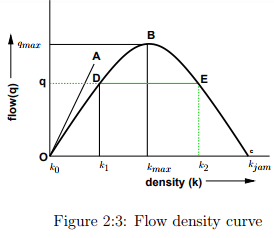The point O refers to the case with zero density and zero flow. The point B refers to the maximum flow and the corresponding density is kmax. The point C refers to the maximum density kjam and the corresponding flow is zero. OA is the tangent drawn to the parabola at O, and the slope of the line OA gives the mean free flow speed, ie the speed with which a vehicle can travel when there is no flow. It can also be noted that points D and E correspond to same flow but has two different densities. Further, the slope of the line OD gives the mean speed at density k1 and slope of the line OE will give mean speed at density k2. Clearly the speed at density k1 will be higher since there are less number of vehicles on the road.

Speed-density diagram

Similar to the flow-density relationship, speed will be maximum, referred to as the free flow speed, and when the density is maximum, the speed will be zero. The most simple assumption is that this variation of speed with density is linear as shown by the solid line in figure 2:4. Corresponding to the zero density, vehicles will be flowing with their desire speed, or free flow speed. When the density is jam density, the speed of the vehicles becomes zero. It is also possible to have non-linear relationships as shown by the dotted lines. These will be discussed later.

Speed flow relation

The relationship between the speed and flow can be postulated as follows. The flow is zero either because there is no vehicles or there are too many vehicles so that they cannot move. At maximum flow, the speed will be in between zero and free flow speed. This relationship is shown in figure 2:5. The maximum flow qmax occurs at speed u. It is possible to have two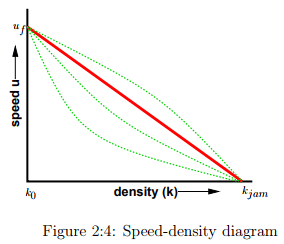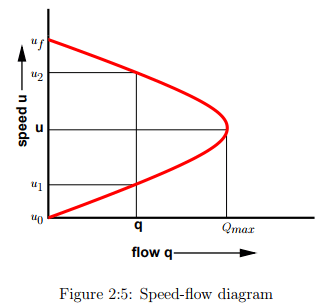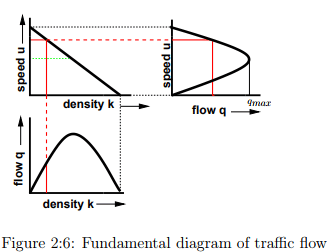different speeds for a given flow.

Combined diagrams

The diagrams shown in the relationship between speed-flow, speed-density, and flow-density are called the fundamental diagrams of traffic flow. These are as shown in figure 2:6. One could observe the inter-relationship of these diagrams.

The document Fundamental Parameters Of Traffic Flow - 2 - Notes | Study Transportation Engineering - Civil Engineering (CE) is a part of the Civil Engineering (CE) Course Transportation Engineering.
All you need of Civil Engineering (CE) at this link: Civil Engineering (CE)

## Transportation Engineering

22 videos|68 docs|39 tests
 Use Code STAYHOME200 and get INR 200 additional OFF

## Transportation Engineering

22 videos|68 docs|39 tests

Track your progress, build streaks, highlight & save important lessons and more!

,

,

,

,

,

,

,

,

,

,

,

,

,

,

,

,

,

,

,

,

,

;